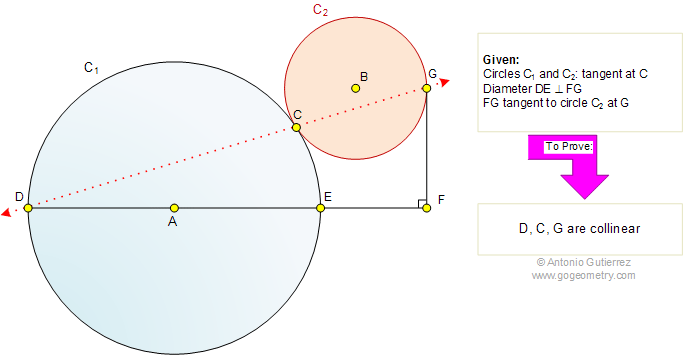Problem 523: Tangent Circles, Diameter Perpendicular, Collinearity. Level: High School, SAT Prep, College geometry

The figure shows circles C1 and C2, tangent at C. Diameter DE extended and FG are perpendicular. If FG is tangent to C2, at G. Prove that the points D, C, and G are collinear. Post a comment or solution.Problem 524.
Circle, Equilateral Triangles, Midpoint, Side, Measurement.

Problem 522.
Right Triangle, Circle, Diameter, Tangent.

Problem 521.
Triangle with three Squares, Altitude, Rectangle, Area.

Problem 520.
Triangle with six Squares, Ratio, Area.

 Home | Search | Geometry | Problems | All Problems | Semicircle | 521-530 | Emai